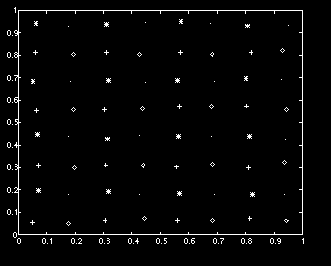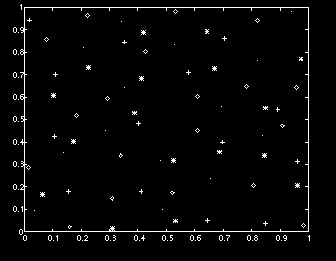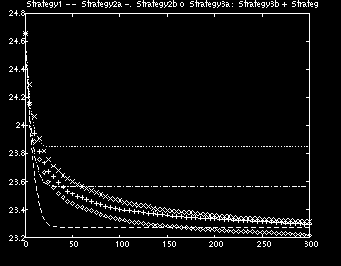Consulting for Ericsson (Sweden)The problem is to assign frequencies to base stations so that the interference among signals sent from the base stations to portable phones is minimized. This problem is known as the frequency assignment problem in the literature and is NP-hard.

We are using two algorithms which approximately minimize the norms of the matrices describing the coupling between base stations to find an approximate solution to this problem. Below there is the solution found by one of our algorithms for a regular distribution of base stations and four frequencies.For more irregular distributions of base stations, the second of our algorithms performs better. Below we show an example.The next graph shows the convergence behavior of our algorithms and their variants.A detailed report is available here:

[Home] [Teaching] [Research] [Consulting] [MathHome] [McGill]